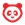# Community

cancel
Showing results for
Did you mean:Community Member

## Multiple assignments in a course that need multiple assignment groups!

Hi! How do I go about setting up Assignment groups when I have say.. 2 assignments with each assignment having different sections with different weight distribution.

So..

I have two assignments in my course. A paper and a video.

Paper is graded: introduction (30%), core (20%) conclusion (50%)

Video is graded: Originality (50%) production quality (50%)

Canvas doesn't seem to have room for grouping different groups in one course.

1 Solution

Accepted SolutionsCommunity Team

I used to teach humanities, and so I graded a ton of papers. If I were teaching this course, I would make a single assignment for "Paper," and weight the three aspects within it through the use of a three-row rubric, assuming a 100-point score for the assignment:

Introduction 30 points
Core 20 points
Conclusion 50 points

I'd do the same thing for the video, but for that assignment the rubric would only have two rows, each one contributing half of the total points (so if the total is 100, each row would be worth 50): Originality and Production Quality.

Is there a separate activity within "Paper" for Introduction, another one for Core, and a third for Conclusion? If so, since Canvas calculates contributions within an assignment group according to their respective points, you could accomplish this by placing the three assignments within a single assignment group for "Paper," and place the three assignment components in it: the Introduction assignment would be worth 30 points, Core is worth 20 points, and Conclusion is worth 50 points.

If the Paper and Video are the only two assignments in the group, you'd only need two assignment groups, and either one of these methods would work, depending on whether the activities are separate or incorporated into a single assignment.Community Team

I used to teach humanities, and so I graded a ton of papers. If I were teaching this course, I would make a single assignment for "Paper," and weight the three aspects within it through the use of a three-row rubric, assuming a 100-point score for the assignment:

Introduction 30 points
Core 20 points
Conclusion 50 points

I'd do the same thing for the video, but for that assignment the rubric would only have two rows, each one contributing half of the total points (so if the total is 100, each row would be worth 50): Originality and Production Quality.

Is there a separate activity within "Paper" for Introduction, another one for Core, and a third for Conclusion? If so, since Canvas calculates contributions within an assignment group according to their respective points, you could accomplish this by placing the three assignments within a single assignment group for "Paper," and place the three assignment components in it: the Introduction assignment would be worth 30 points, Core is worth 20 points, and Conclusion is worth 50 points.

If the Paper and Video are the only two assignments in the group, you'd only need two assignment groups, and either one of these methods would work, depending on whether the activities are separate or incorporated into a single assignment.